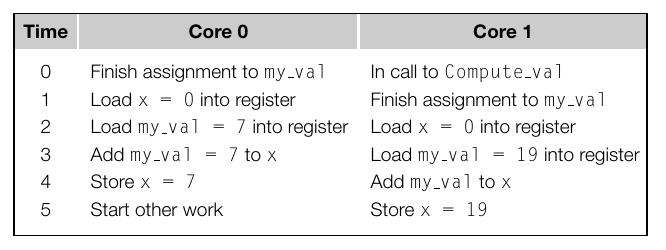# Parallel Software

• Share data implicitly.
• Must protect data explicitly.
• Can only be used on shared memory systems.

The number of threads grows and shrinks as the amount of work varies. The main thread spawns new threads to handle tasks. Often used for server applications.

The number of threads is constant throughout the running of the program. Threads can either have a fixed amount of work, or be given tasks dynamically.

## Non-Determinism

Non-Determinism means that a program can have different results with the same input. Multi-threaded programs often suffer from non-determinism.

Suppose two threads execute the following printf call:




The output could be:

Thread 0: value = 7.


Or:

Thread 1: value = 19.


Or worse:

Thread Thread 1: val0:value = 7.
ue = 19.


This example demonstrates this.


my_val = Compute_val();
x += my_val;


This may work as expected, but it may not. Statements in C are typically not atomic. It may happen as follows:Non-determinism makes parallel programs difficult to debug.

## Terminology

Bugs like the above are called race conditions.

A piece of code that contains a race condition is called a critical section

In multi-threaded programs, critical sections must be handled so that only one thread is in the critical section at a time.

This is called mutual exclusion. It can be implemented with a mutual exclusion lock, normally called a mutex or a lock.

## Mutual Exclusion

Multi-threading systems have mutex locks built in. We can fix the incorrect program above as follows:


my_val = Compute_val();

Lock(&my_val_lock);
x += my_val;
Unlock(&my_val_lock);


Locks can slow down a program severely if not used carefully.

Mutexes give us the ability to fix non-determinism in our own code. We can also run into issues with calling library code. Libraries which can be invoked safely from multiple threads are thread safe.

Some libraries are not thread safe. For example, strtok, a C function for splitting strings.


#include <string.h>
#include <stdio.h>

int main() {
const char str [] = "Some text we want to split up";
char* token;

/* get the first token */
token = strtok(str, " ");

/* walk through other tokens */
while (token != NULL) {
printf("%s\n", token);

token = strtok(NULL, " ");
}

return(0);
}


strtok works by keeping a global variable pointing into the string you first pass it. If strtok is called from multiple threads, they will trash each other's state.

## Multi-Process Programming

Multi-Process programs:
• Must share data explicitly.
• Protect data implicitly.
• Can be run on shared memory or distributed memory systems.
• Processes can communicate via message passing, or pipes.

## Message Passing

Message passing is a common way for multi-process programs to communicate. This is done with "send" and "receive" functions. Processes are identified by "rank":


char message;

my_rank = Getrank();

/* processor 1 sends a message */
if (my_rank == 1) {
strcpy(message, "Greetings from processor 1!");
Send(message, MSG_CHAR, 100, 0);

/* processor 0 receives a message */
} else if (my_rank == 0) {
printf("Processor 0 received message '%s'\n", message);
}


• The "Receive" function usually blocks, MPI also allows us to check if a message is ready for us.
• MPI also contains a broadcast facility where we can send a message to all processes.

## Multiple Threads vs. Multiple Processes

• Threads make sharing easier and protecting harder.
• Processes make sharing harder and protecting easier.
• It's typically easier to parallelize existing applications with threads.

## Input & Output

Input and output is non-deterministic in parallel programs. What should happen if two threads call scanf at the same time?

Generally, only one thread should perform I/O (sometimes only one thread is allowed to access stdin).

Debugging output statements are a good idea, however.

## Speedup & Efficiency

Speedup is defined as follows:

$S = \frac{T_{serial}}{T_{parallel}}$

Ideally we would get linear speedup meaning that the speedup is equal to the number of cores working on the problem.

In practice, this does not happen due to communication overhead.

We can also measure the efficiency of our program:

$E = \frac{S}{P}$

Efficiency is the speedup we get per-core, it reflects how close our speedup is to the ideal case of linear.

If we have a serial program that runs in 18 minutes, and a parallel program that runs in 2.5 minutes on 8 cores, what is the speedup and efficiency?

## Amdahl's Law

Parallel programs typically consist of serial and parallel portions. The serial portion of a program limits the amount of speedup we can achieve.

If B is the fraction of our program that is strictly serial, and the rest can be parallelized, Amdahl's law says that the time taken to execute our program on $P$ cores is given by the function:

$T(P) = T(1) * (B + \frac{1}{P}(1 - B))$

For example, if:

• We have a program that takes 10 minutes to run on one core.
• We have an 8-core machine to run it on.
• 80% of the program can be done in parallel.

How fast will it run in the ideal case?

Our speedup under Amdahl's law can be given as:

$S(P) = \frac{T(1)}{T(P)} = \frac{T(1)}{T(1) * (B + \frac{1}{P}(1 - B))} = \frac{1}{B + \frac{1}{P}(1 - B)}$

In the example above, what speedup can we expect?

Given 8 processors, the table below shows the speedup we can expect with different values of B:

 Serial Fraction Expected Speedup 0.0 8.0 0.05 5.9 0.1 4.7 0.2 3.3 0.3 2.6 0.4 2.1 0.5 1.8

The amount of code we must run sequentially greatly affects the speedup we can expect.

Amdahl's law also limits the usefulness of adding more processors to program:The amount of serial code in our programs greatly affects performance!